Скачать презентацию How Much Information Is In A Quantum State

• Количество слайдов: 27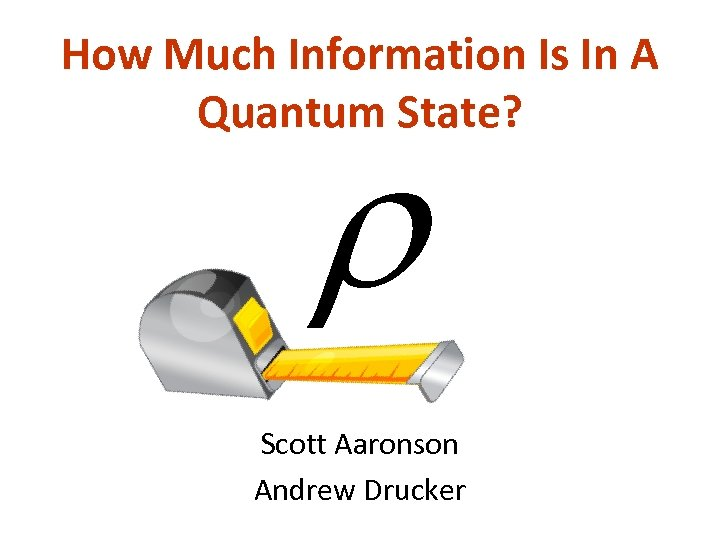How Much Information Is In A Quantum State? Scott Aaronson Andrew Drucker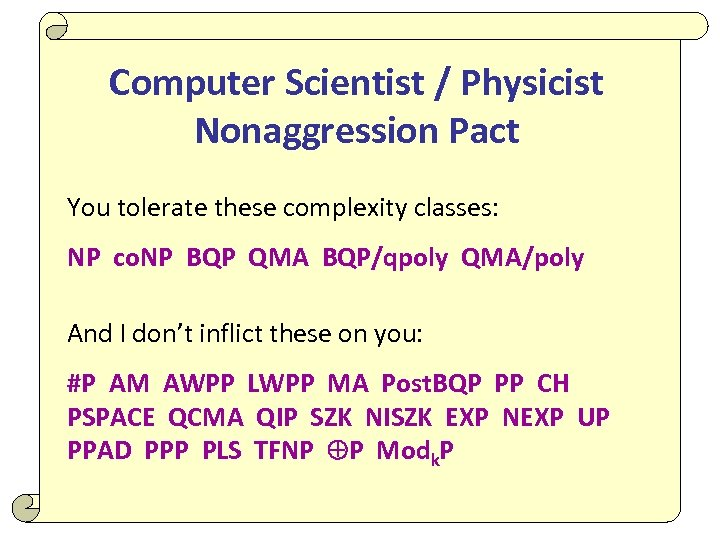Computer Scientist / Physicist Nonaggression Pact You tolerate these complexity classes: NP co. NP BQP QMA BQP/qpoly QMA/poly And I don’t inflict these on you: #P AM AWPP LWPP MA Post. BQP PP CH PSPACE QCMA QIP SZK NISZK EXP NEXP UP PPAD PPP PLS TFNP P Modk. P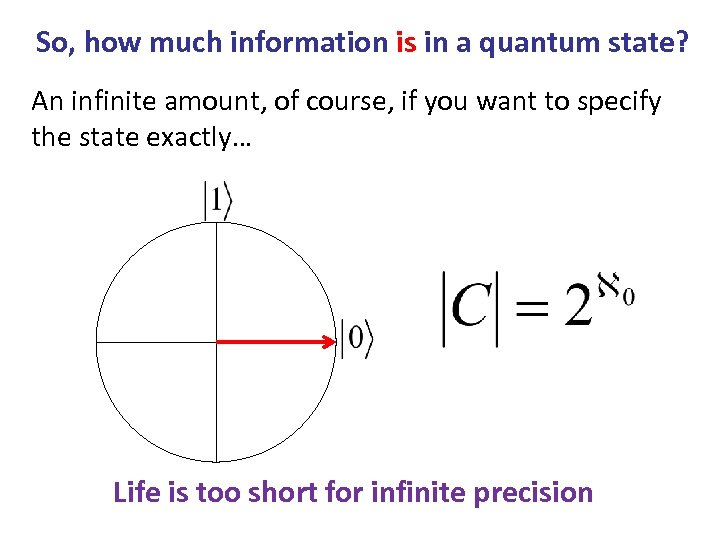So, how much information is in a quantum state? An infinite amount, of course, if you want to specify the state exactly… Life is too short for infinite precision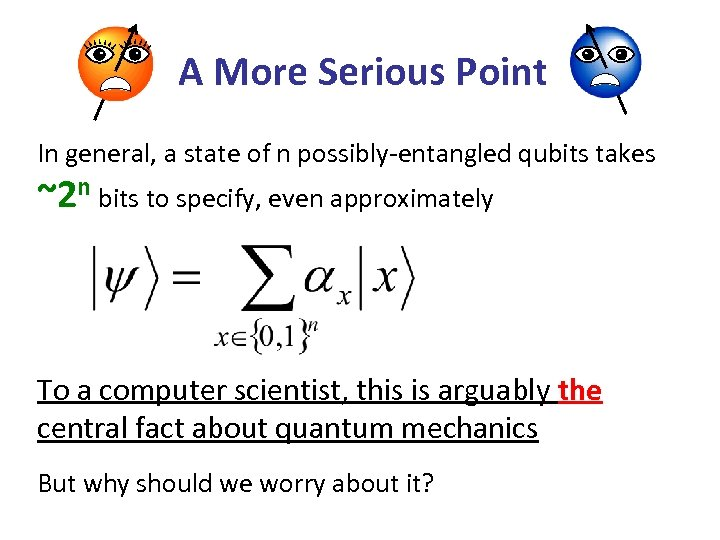A More Serious Point In general, a state of n possibly-entangled qubits takes ~2 n bits to specify, even approximately To a computer scientist, this is arguably the central fact about quantum mechanics But why should we worry about it?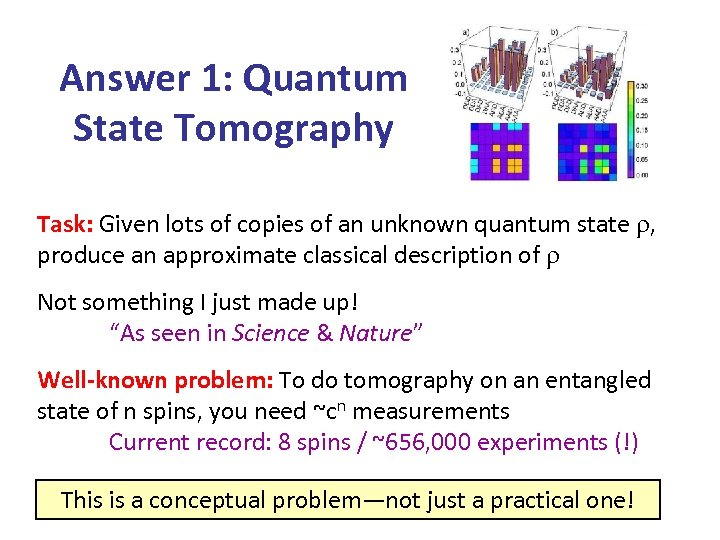Answer 1: Quantum State Tomography Task: Given lots of copies of an unknown quantum state , produce an approximate classical description of Not something I just made up! “As seen in Science & Nature” Well-known problem: To do tomography on an entangled state of n spins, you need ~cn measurements Current record: 8 spins / ~656, 000 experiments (!) This is a conceptual problem—not just a practical one!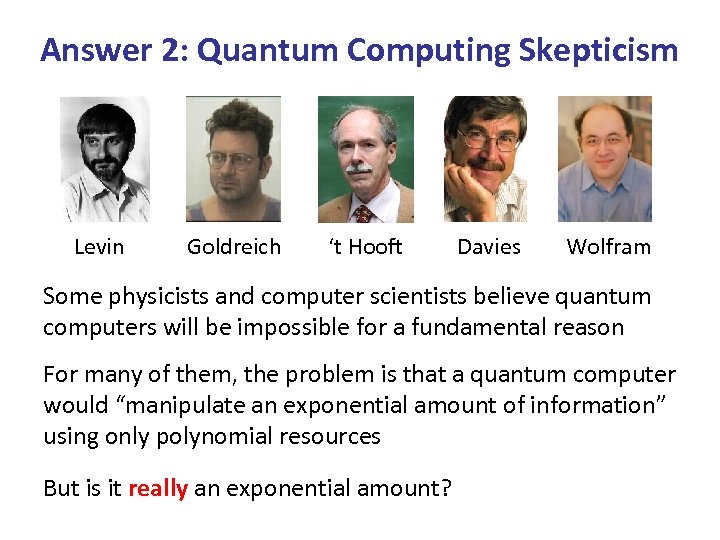Answer 2: Quantum Computing Skepticism Levin Goldreich ‘t Hooft Davies Wolfram Some physicists and computer scientists believe quantum computers will be impossible for a fundamental reason For many of them, the problem is that a quantum computer would “manipulate an exponential amount of information” using only polynomial resources But is it really an exponential amount?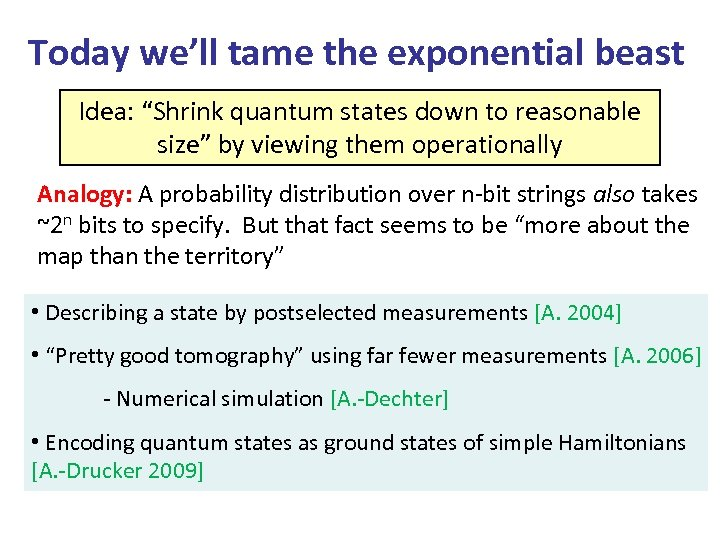Today we’ll tame the exponential beast Idea: “Shrink quantum states down to reasonable size” by viewing them operationally Analogy: A probability distribution over n-bit strings also takes ~2 n bits to specify. But that fact seems to be “more about the map than the territory” • Describing a state by postselected measurements [A. 2004] • “Pretty good tomography” using far fewer measurements [A. 2006] - Numerical simulation [A. -Dechter] • Encoding quantum states as ground states of simple Hamiltonians [A. -Drucker 2009]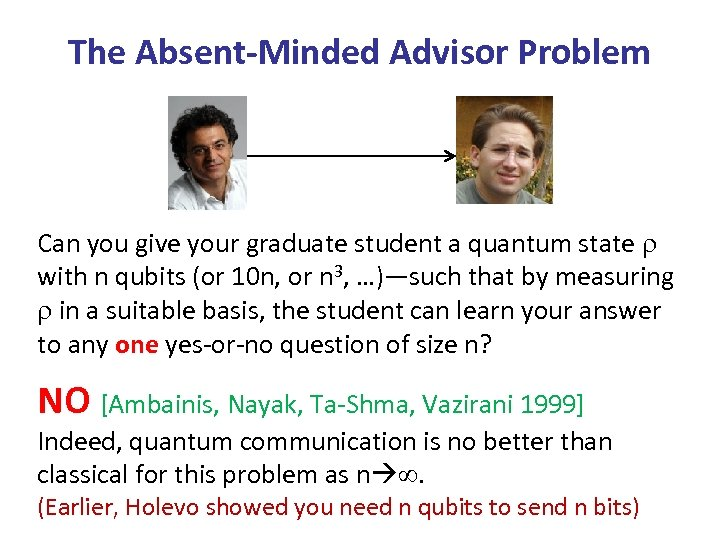The Absent-Minded Advisor Problem Can you give your graduate student a quantum state with n qubits (or 10 n, or n 3, …)—such that by measuring in a suitable basis, the student can learn your answer to any one yes-or-no question of size n? NO [Ambainis, Nayak, Ta-Shma, Vazirani 1999] Indeed, quantum communication is no better than classical for this problem as n . (Earlier, Holevo showed you need n qubits to send n bits)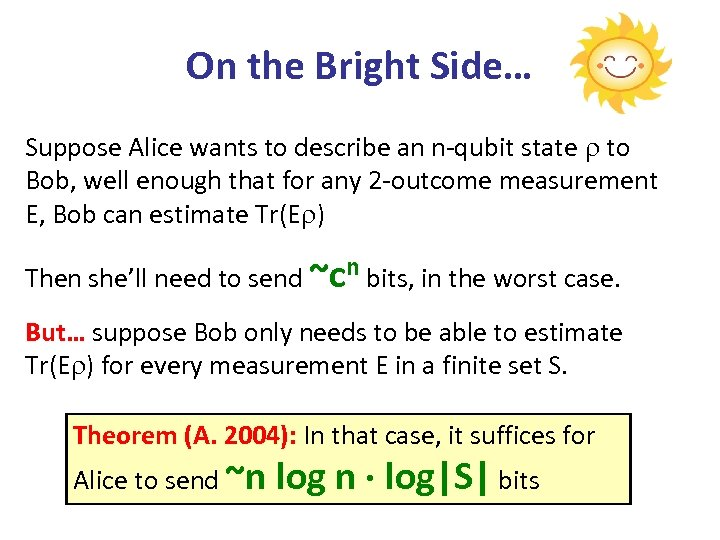On the Bright Side… Suppose Alice wants to describe an n-qubit state to Bob, well enough that for any 2 -outcome measurement E, Bob can estimate Tr(E ) Then she’ll need to send ~cn bits, in the worst case. But… suppose Bob only needs to be able to estimate Tr(E ) for every measurement E in a finite set S. Theorem (A. 2004): In that case, it suffices for Alice to send ~n log n log|S| bits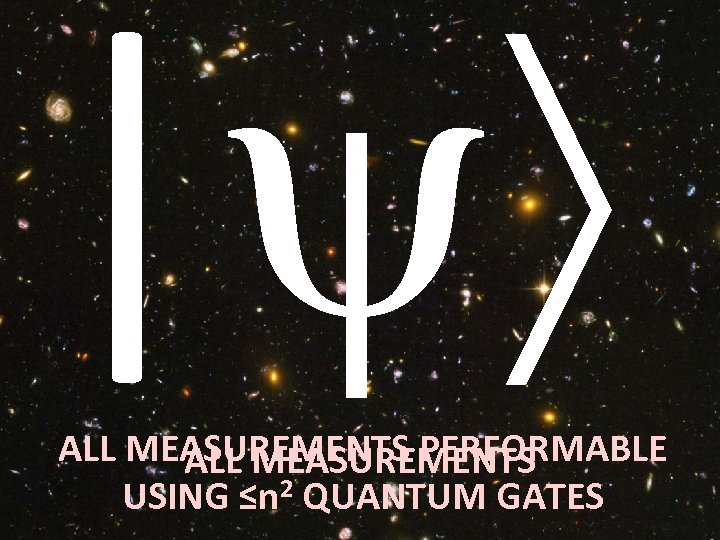| ALL MEASUREMENTS PERFORMABLE ALL MEASUREMENTS USING ≤n 2 QUANTUM GATES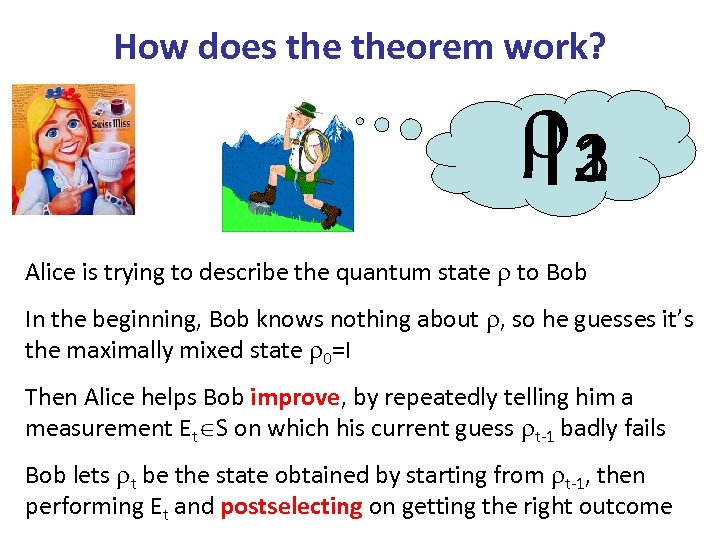How does theorem work? 1 3 I 2 Alice is trying to describe the quantum state to Bob In the beginning, Bob knows nothing about , so he guesses it’s the maximally mixed state 0=I Then Alice helps Bob improve, by repeatedly telling him a measurement Et S on which his current guess t-1 badly fails Bob lets t be the state obtained by starting from t-1, then performing Et and postselecting on getting the right outcome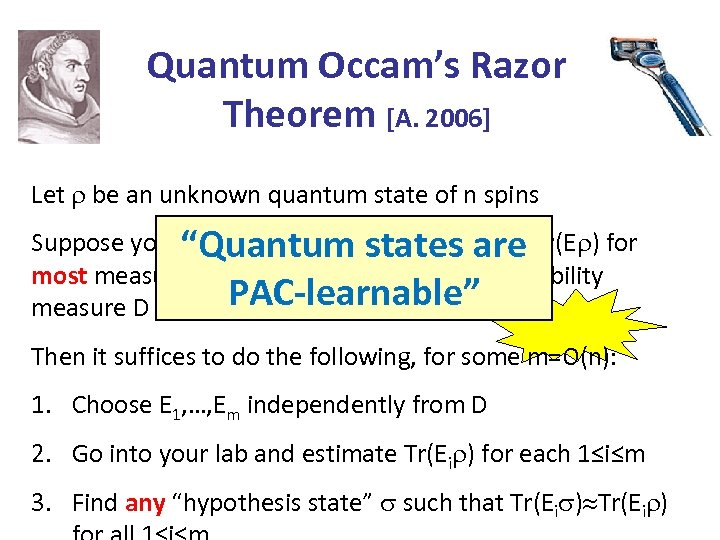Quantum Occam’s Razor Theorem [A. 2006] Let be an unknown quantum state of n spins Suppose you just want to be able to estimate Tr(E ) for “Quantum states are most measurements E drawn from some probability PAC-learnable” measure D Then it suffices to do the following, for some m=O(n): 1. Choose E 1, …, Em independently from D 2. Go into your lab and estimate Tr(Ei ) for each 1≤i≤m 3. Find any “hypothesis state” such that Tr(Ei )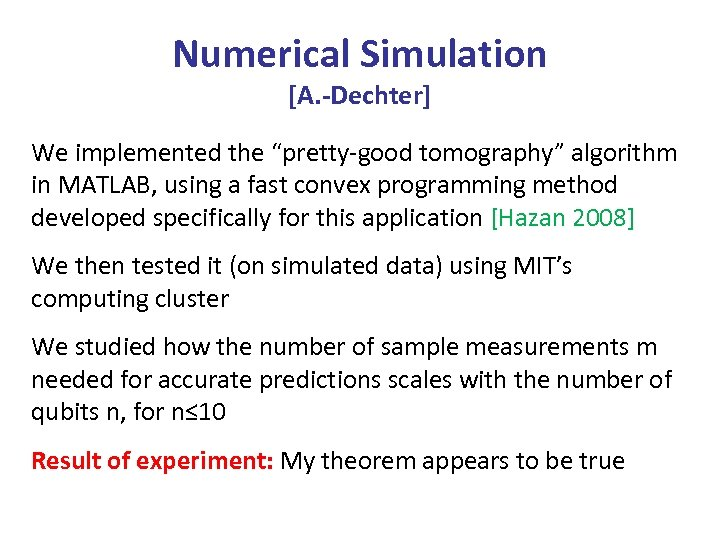Numerical Simulation [A. -Dechter] We implemented the “pretty-good tomography” algorithm in MATLAB, using a fast convex programming method developed specifically for this application [Hazan 2008] We then tested it (on simulated data) using MIT’s computing cluster We studied how the number of sample measurements m needed for accurate predictions scales with the number of qubits n, for n≤ 10 Result of experiment: My theorem appears to be true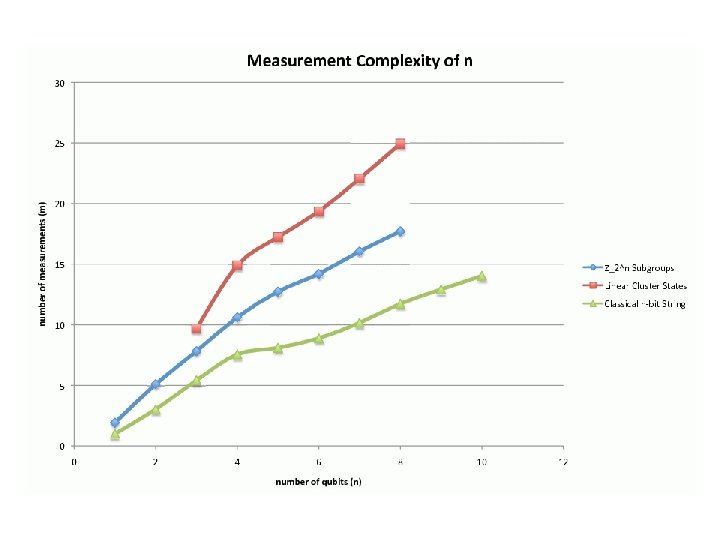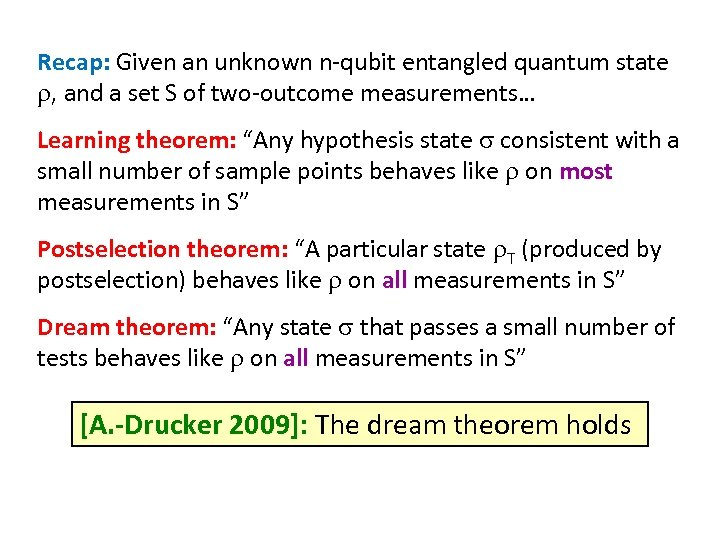Recap: Given an unknown n-qubit entangled quantum state , and a set S of two-outcome measurements… Learning theorem: “Any hypothesis state consistent with a small number of sample points behaves like on most measurements in S” Postselection theorem: “A particular state T (produced by postselection) behaves like on all measurements in S” Dream theorem: “Any state that passes a small number of tests behaves like on all measurements in S” [A. -Drucker 2009]: The dream theorem holds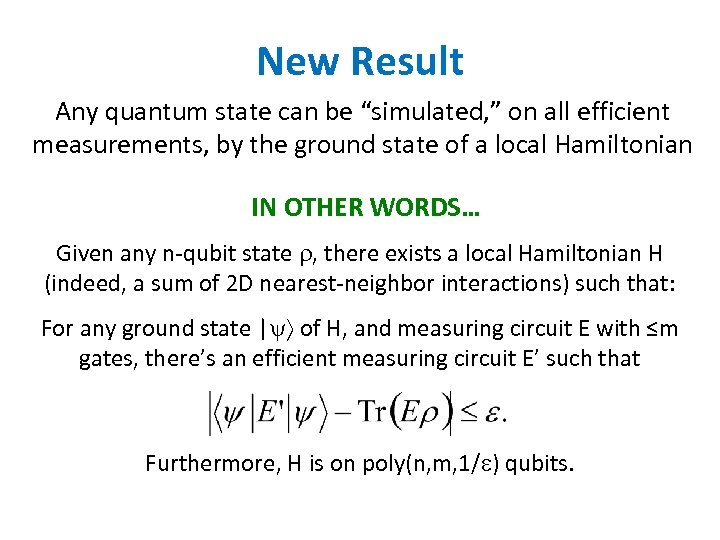New Result Any quantum state can be “simulated, ” on all efficient measurements, by the ground state of a local Hamiltonian IN OTHER WORDS… Given any n-qubit state , there exists a local Hamiltonian H (indeed, a sum of 2 D nearest-neighbor interactions) such that: For any ground state | of H, and measuring circuit E with ≤m gates, there’s an efficient measuring circuit E’ such that Furthermore, H is on poly(n, m, 1/ ) qubits.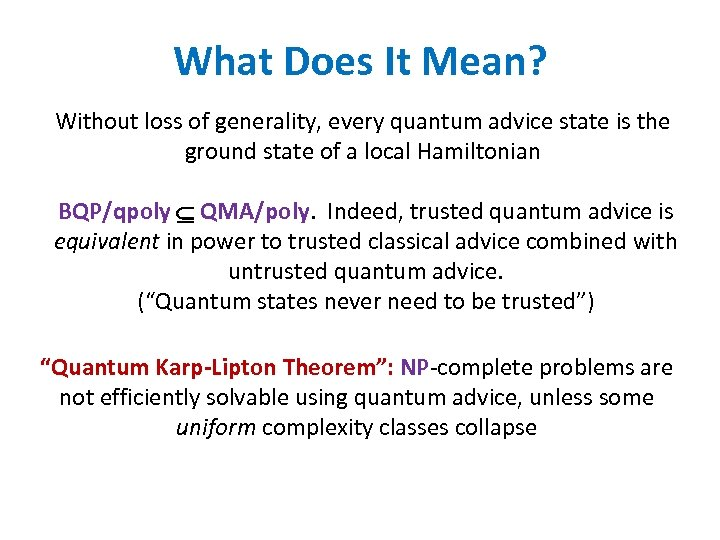What Does It Mean? Without loss of generality, every quantum advice state is the ground state of a local Hamiltonian BQP/qpoly QMA/poly. Indeed, trusted quantum advice is equivalent in power to trusted classical advice combined with untrusted quantum advice. (“Quantum states never need to be trusted”) “Quantum Karp-Lipton Theorem”: NP-complete problems are not efficiently solvable using quantum advice, unless some uniform complexity classes collapse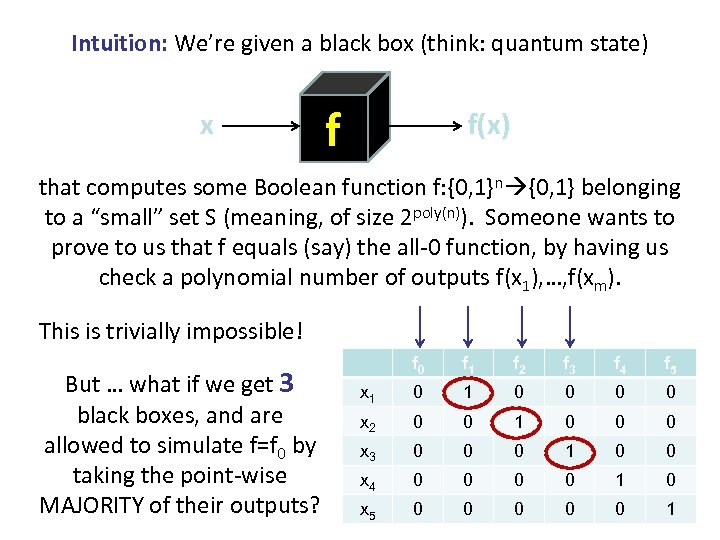Intuition: We’re given a black box (think: quantum state) x f f(x) that computes some Boolean function f: {0, 1}n {0, 1} belonging to a “small” set S (meaning, of size 2 poly(n)). Someone wants to prove to us that f equals (say) the all-0 function, by having us check a polynomial number of outputs f(x 1), …, f(xm). This is trivially impossible! But … what if we get 3 black boxes, and are allowed to simulate f=f 0 by taking the point-wise MAJORITY of their outputs? f 0 f 1 f 2 f 3 f 4 f 5 x 1 0 0 0 0 x 2 0 0 1 0 0 0 x 3 0 0 0 1 0 0 x 4 0 0 1 0 x 5 0 0 0 1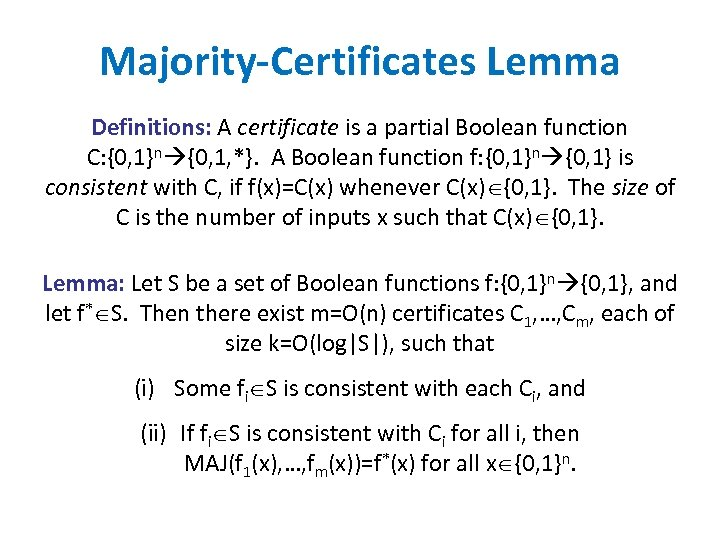Majority-Certificates Lemma Definitions: A certificate is a partial Boolean function C: {0, 1}n {0, 1, *}. A Boolean function f: {0, 1}n {0, 1} is consistent with C, if f(x)=C(x) whenever C(x) {0, 1}. The size of C is the number of inputs x such that C(x) {0, 1}. Lemma: Let S be a set of Boolean functions f: {0, 1}n {0, 1}, and let f* S. Then there exist m=O(n) certificates C 1, …, Cm, each of size k=O(log|S|), such that (i) Some fi S is consistent with each Ci, and (ii) If fi S is consistent with Ci for all i, then MAJ(f 1(x), …, fm(x))=f*(x) for all x {0, 1}n.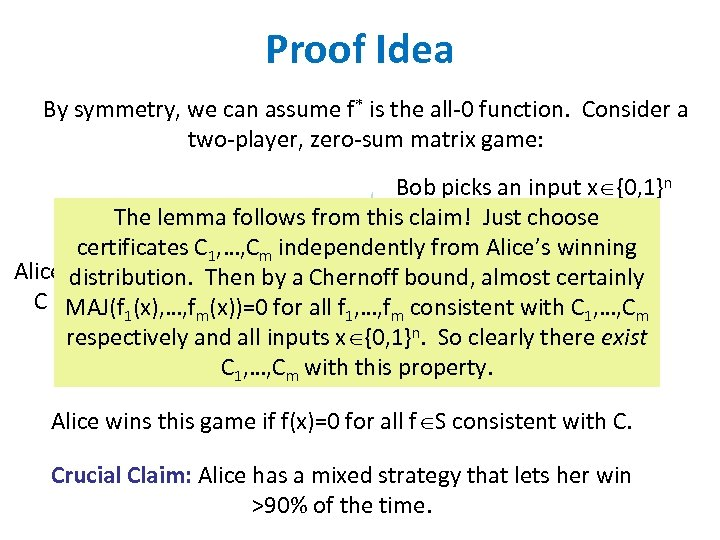Proof Idea By symmetry, we can assume f* is the all-0 function. Consider a two-player, zero-sum matrix game: Bob picks an input x {0, 1}n The lemma follows from this claim! Just choose certificates C 1, …, Cm independently from Alice’s winning Alice picks a certificate by a Chernoff bound, almost certainly distribution. Then C of size k(x), …, fm(x))=0 for all f 1, …, fm consistent with C 1, …, Cm MAJ(f 1 consistent with some f S respectively and all inputs x {0, 1}n. So clearly there exist C 1, …, Cm with this property. Alice wins this game if f(x)=0 for all f S consistent with C. Crucial Claim: Alice has a mixed strategy that lets her win >90% of the time.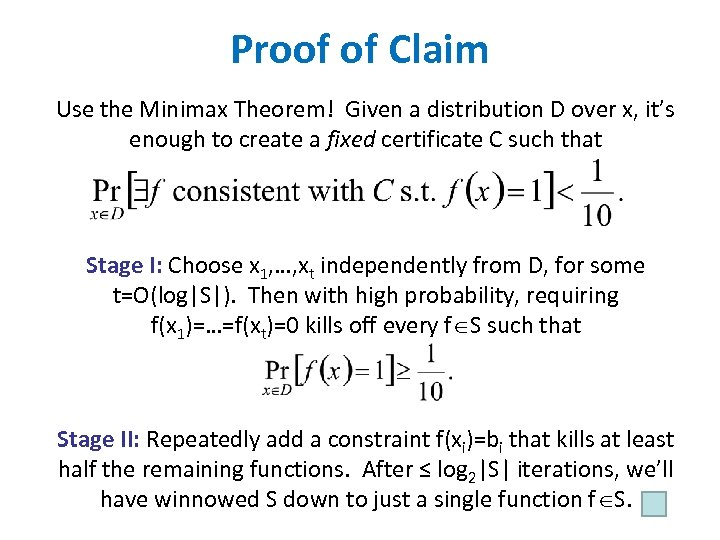Proof of Claim Use the Minimax Theorem! Given a distribution D over x, it’s enough to create a fixed certificate C such that Stage I: Choose x 1, …, xt independently from D, for some t=O(log|S|). Then with high probability, requiring f(x 1)=…=f(xt)=0 kills off every f S such that Stage II: Repeatedly add a constraint f(xi)=bi that kills at least half the remaining functions. After ≤ log 2|S| iterations, we’ll have winnowed S down to just a single function f S.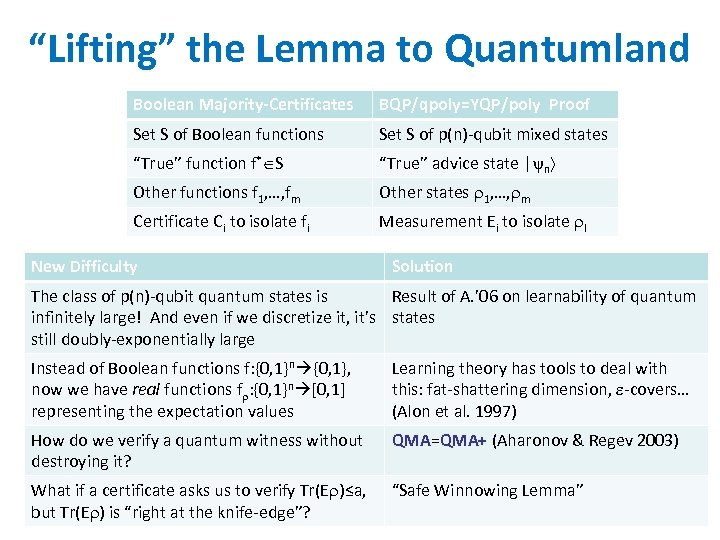“Lifting” the Lemma to Quantumland Boolean Majority-Certificates BQP/qpoly=YQP/poly Proof Set S of Boolean functions Set S of p(n)-qubit mixed states “True” function f* S “True” advice state | n Other functions f 1, …, fm Other states 1, …, m Certificate Ci to isolate fi Measurement Ei to isolate I New Difficulty Solution The class of p(n)-qubit quantum states is Result of A. ’ 06 on learnability of quantum infinitely large! And even if we discretize it, it’s states still doubly-exponentially large Instead of Boolean functions f: {0, 1}n {0, 1}, now we have real functions f : {0, 1}n [0, 1] representing the expectation values Learning theory has tools to deal with this: fat-shattering dimension, -covers… (Alon et al. 1997) How do we verify a quantum witness without destroying it? QMA=QMA+ (Aharonov & Regev 2003) What if a certificate asks us to verify Tr(E )≤a, but Tr(E ) is “right at the knife-edge”? “Safe Winnowing Lemma”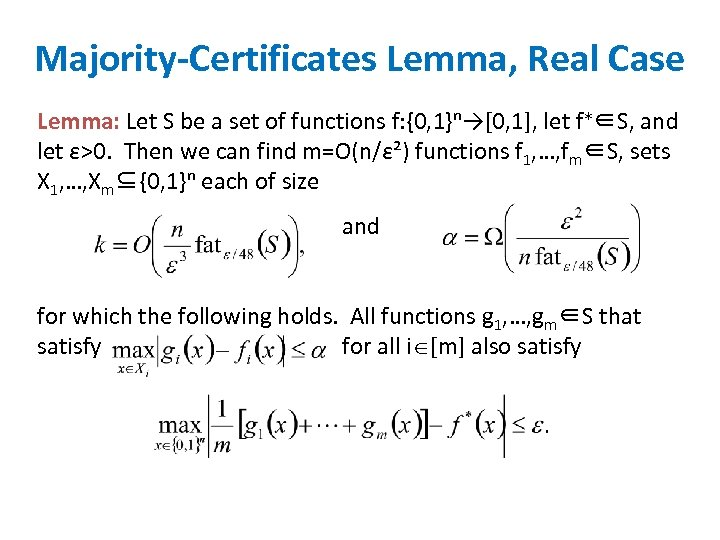Majority-Certificates Lemma, Real Case Lemma: Let S be a set of functions f: {0, 1}ⁿ→[0, 1], let f∗∈S, and let ε>0. Then we can find m=O(n/ε²) functions f 1, …, fm∈S, sets X 1, …, Xm⊆{0, 1}ⁿ each of size and for which the following holds. All functions g 1, …, gm∈S that satisfy for all i [m] also satisfy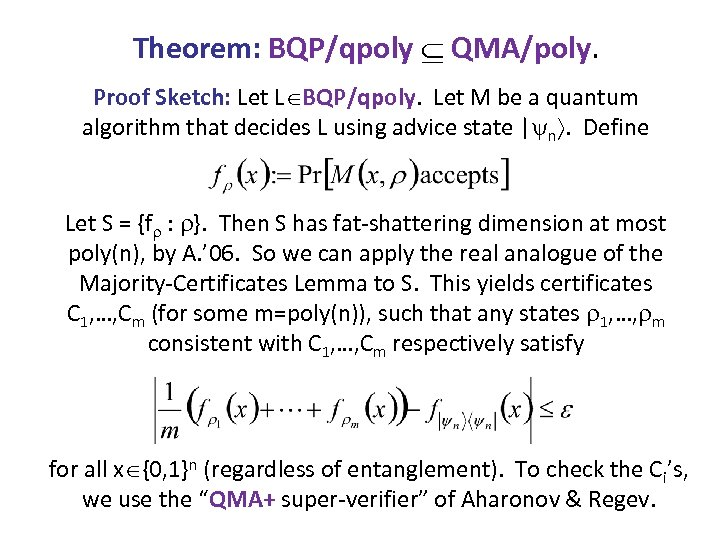Theorem: BQP/qpoly QMA/poly. Proof Sketch: Let L BQP/qpoly. Let M be a quantum algorithm that decides L using advice state | n. Define Let S = {f : }. Then S has fat-shattering dimension at most poly(n), by A. ’ 06. So we can apply the real analogue of the Majority-Certificates Lemma to S. This yields certificates C 1, …, Cm (for some m=poly(n)), such that any states 1, …, m consistent with C 1, …, Cm respectively satisfy for all x {0, 1}n (regardless of entanglement). To check the Ci’s, we use the “QMA+ super-verifier” of Aharonov & Regev.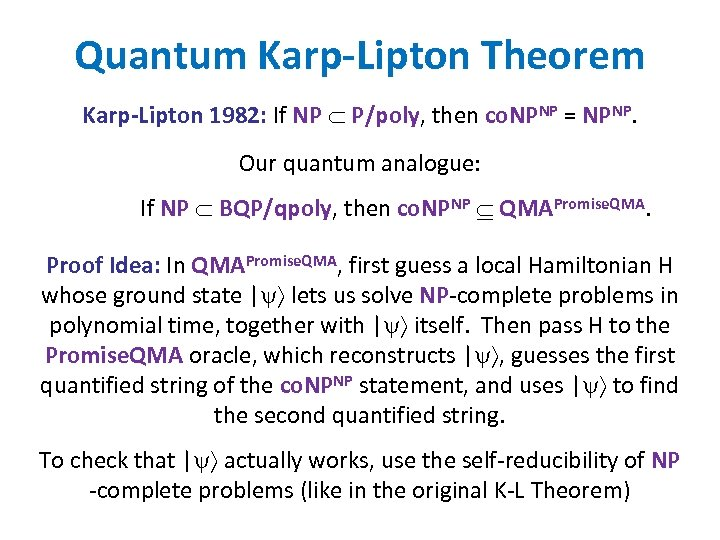Quantum Karp-Lipton Theorem Karp-Lipton 1982: If NP P/poly, then co. NPNP = NPNP. Our quantum analogue: If NP BQP/qpoly, then co. NPNP QMAPromise. QMA. Proof Idea: In QMAPromise. QMA, first guess a local Hamiltonian H whose ground state | lets us solve NP-complete problems in polynomial time, together with | itself. Then pass H to the Promise. QMA oracle, which reconstructs | , guesses the first quantified string of the co. NPNP statement, and uses | to find the second quantified string. To check that | actually works, use the self-reducibility of NP -complete problems (like in the original K-L Theorem)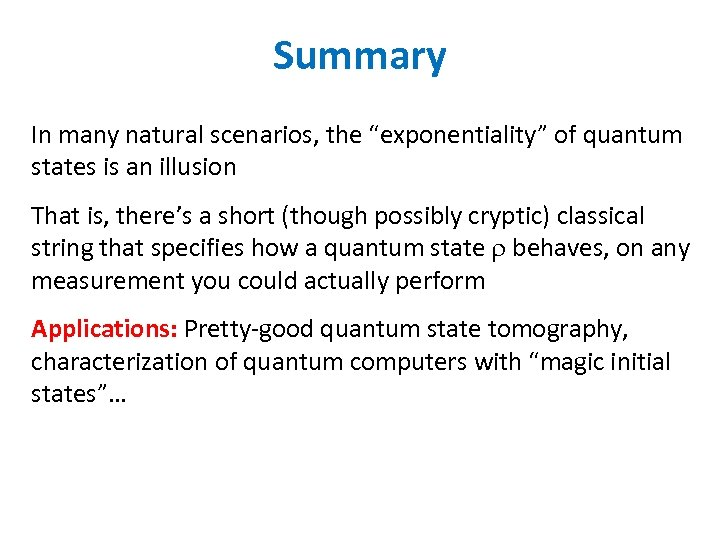Summary In many natural scenarios, the “exponentiality” of quantum states is an illusion That is, there’s a short (though possibly cryptic) classical string that specifies how a quantum state behaves, on any measurement you could actually perform Applications: Pretty-good quantum state tomography, characterization of quantum computers with “magic initial states”…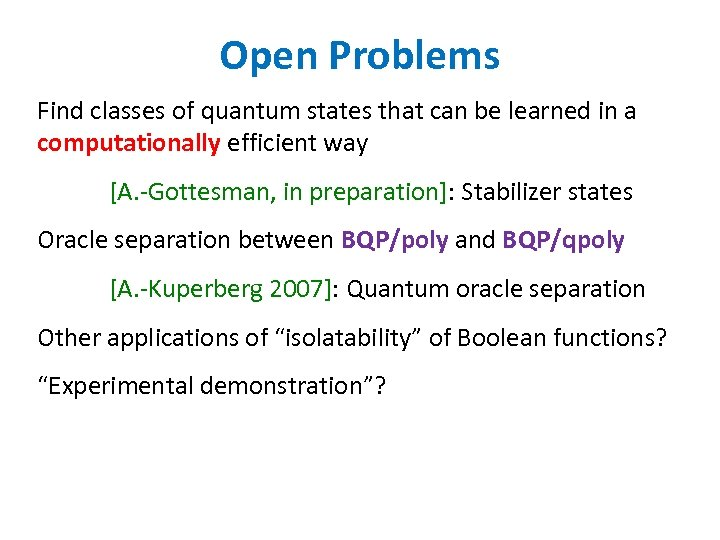Open Problems Find classes of quantum states that can be learned in a computationally efficient way [A. -Gottesman, in preparation]: Stabilizer states Oracle separation between BQP/poly and BQP/qpoly [A. -Kuperberg 2007]: Quantum oracle separation Other applications of “isolatability” of Boolean functions? “Experimental demonstration”?